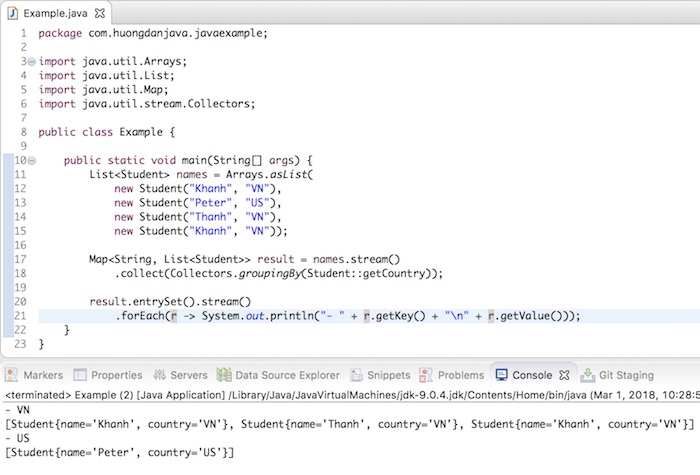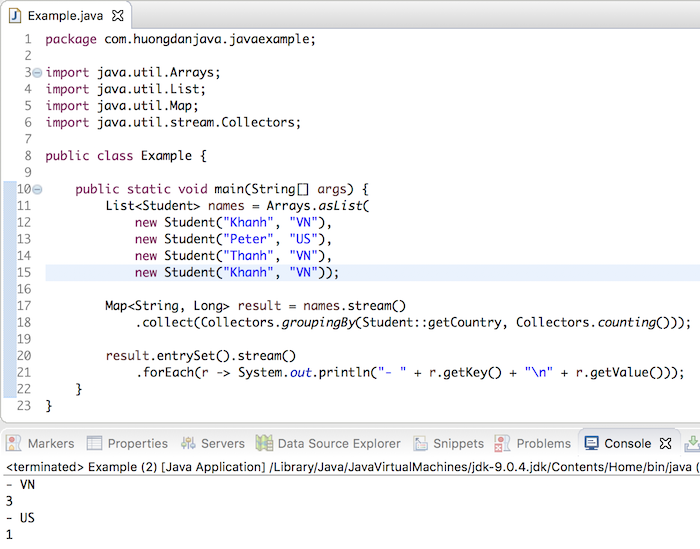# Group by using Stream and Collectors in Java

In SQL, the GROUP BY statement returns the set of records having the same value in particular criteria. For example, you have a table that contains student information, each student coming from a different country. Use the GROUP BY statement according to national criteria, you can know the number of students from some countries. Similarly, from version 8, Java also supports us by the group by function. In this tutorial, I will show you how to group by using Stream and Collectors in Java.

For example, I have a list of students with the following information:

Now, I need to group the above student list by country and count the number of students in each country.

To do this, we will use the collect() method in the Stream object with the groupingBy() static method in the Java Collectors object.

For example, if we need a group of students by country, we would like to code as follows:

Result:In the code above, the parameter of the groupingBy() method is the same as the criteria in the SQL. If you want to group by student name then you can replace Student::getCountry with Student::getName.

As you can see, by default after the group is finished, any element that has the same criteria falls into a List object, we can change this by passing another parameter to the groupingBy() method of the Collectors object. For example, now that I want the elements with the same criteria to be in a Set object, I would like to code as follows:

Result:Also, if after grouping, you want to count the number of students in each country, then use the groupingBy method as follows:

Here, I used another method of the Collectors object called counting() to count the number of students in each country that I wanted.

Result: